# Candies

If Alena give Lenka 3 candy will still have 1 more candy. If Lenka give Alena 1 candy Alena will hame twice more than Lenka. How many candies have each of them?

Correct result:

a =  17
l =  10

#### Solution:

a-3 = l+3+1
2(l-1) = a+1

a-3 = l+3+1
2•(l-1) = a+1

a-l = 7
a-2l = -3

a = 17
l = 10

Our linear equations calculator calculates it.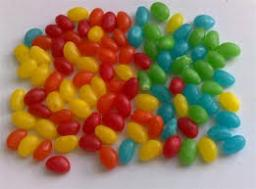We would be pleased if you find an error in the word problem, spelling mistakes, or inaccuracies and send it to us. Thank you!Tips to related online calculators
Do you have a system of equations and looking for calculator system of linear equations?

## Next similar math problems:

• CandyPeter had a sachet of candy. He wanted to share with his friends. If he gave them 30 candies, he would have 62 candies. If he gave them 40 candies, he would miss 8 candies. How many friends did Peter have?
• AntennasIf you give me two antennas will be same. If you give me again your two antenna I have a 5× so many than you. How many antennas have both mans?
• Balls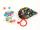Michal said to Martin: give me one ball and I'll have twice as you. Martin said: give me 4 and we will have equally. How many balls each have?
• Sweets, candyGrandfather gave out sweets to four children. At the last moment, two more children came, so in order to have them all the same, each of the four children would receive four candies less than they would have received if they had not. How much did my grand
• Candy and boxesWe have some number of candy and empty boxes. When we put candies in boxes of ten, there will be 2 candies and 8 empty boxes left, when of eight, there will be 6 candies and 3 boxes left. How many candy and empty boxes left when we put candies in boxes of
• Three friends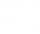Peter is eight times older than Rado, Rado is 3 times younger than Joseph. Together they have 120 years. Determine how many are each of them.
• 3 masons3 masons received 7,700 CZK. The second half received 1/2 more than the first and third twice more than the second mason. How much they each got crowns?
• PearsAndrew, Lenka and Rasto have together 232 pears. Lenka has 28 more than Rasto and Rasto pears have 96 more than Andrew. Determine how much each of them has pears.
• Division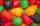Three siblings Helena, Oliver and George split the bag with candies on merit in the ratio 6:1:4. How many candies should each get if in bag were 88?
• Three workersThree workers were rewarded CZK 9200 and the money divided by the work they have done. First worker to get twice than the second, the second three times more than the third. How much money each worker received?
• GrandmotherGrandmother wants to give the candies to grandchildren that when she gives 5 candy everyone 3 missing and when she gives 4 candies 3 is surplus. How many grandchildren has grandmother and how many candies has?
• Boxes200 boxes have been straightened in three rows. The first was 13 more than in the second, and in the second was one fifth more than in the third one. How many boxes are in each row?
• Three friends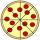Divide 570 euros to three friends so that first will get 50 euros less than the second and third twice more than the first. How many euros will get everyone?
• Three friendsThe three friends spent 600 KC in a teahouse. Thomas paid twice as much as Paul. Paul a half less than Zdeněk. How many each paid?
• Euler problem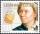Someone buys a 180 tolars towels. If it was for the same money of 3 more towels, it would be 3 tolars cheaper each. How many were towels?
• Three daysDuring the three days sold in stationery 1490 workbooks. The first day sold about workbooks more than third day. The second day 190 workbooks sold less than third day. How many workbooks sold during each day?
• Box of chocolatesIn a box of chocolates was 16 candies. Christopher and Luke they were distributed: a) Christopher had about 4 candies over Luke, b) Christopher had about 6 candies less than Luke, c) Christopher had 3 times more sweet than Luke. How many had each boy cand